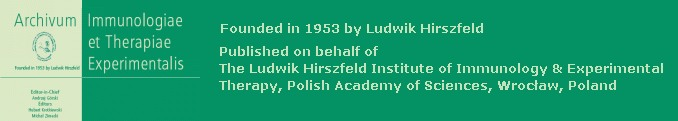# AITE Impact Factor (IF)Impact factor of AITE, calculated for the year 2020, was 4.291.
Impact factor of AITE, calculated for the year 2019, was 3.200.
Impact factor of AITE, calculated for the year 2018, was 2.878.
Impact factor of AITE, calculated for the year 2017, was 2.991.
Impact factor of AITE, calculated for the year 2016, was 2.040.
Impact factor of AITE, calculated for the year 2015, was 2.464.
Impact factor of AITE, calculated for the year 2014, was 3.176.
Impact factor of AITE, calculated for the year 2013, was 2.818.
Impact factor of AITE, calculated for the year 2012, was 2.378.
Impact factor of AITE, calculated for the year 2011, was 2.541.
Impact factor of AITE, calculated for the year 2010, was 2.385.
Impact factor of AITE, calculated for the year 2009, was 1.989.
Impact factor of AITE, calculated for the year 2008, was 1.432.
Impact factor of AITE, calculated for the year 2007, was 1.689.
Impact factor of AITE, calculated for the year 2006, was 1.635.
Impact factor of AITE, calculated for the year 2005, was 1.000.
Impact factor of AITE, calculated for the year 2004, was 0.663.
Impact factor of AITE, calculated for the year 2003, was 0.707.

Archivum Immunologiae et Therapiae Experimentalis enters the ISI Master Journal List (Thomson ISI, Philadelphia, USA) with Impact Factor (2002) = 0.793 (ISI estimate)

Impact factor of AITE, calculated for the year 2001, was 0.587.
Impact factor of AITE, calculated for the year 2000, was 0.413.

Self-citation rates:
2020 – 1.6%
2019 – 1.2%
2018 – 0.3%
2017 – 2.5%
2016 – 1.5%
2015 – 1.2%
2014 – 1.0%
2013 – 1.6%
2012 – 1,4%
2011 – 1.2%
2010 – 1.3%
2009 – 2.7%
2008 – 4.4%
2007 – 6.0%
2006 – 4.0%
2005 – 6.1%
2004 – 5.4%
2003 – 3.7%

“a self-citation rate of 20% or less is characteristic of the majority of the high-quality science journals selected for coverage…” (a mean self-citation rate = 12.41, with a median of 9.04)
http://scientific.thomsonreuters.com/free/essays/journalcitationreports/selfcitation2002/